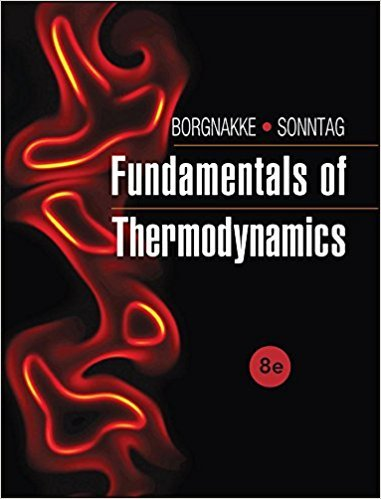×
Get Full Access to Fundamentals Of Thermodynamcs - 8 Edition - Chapter 1 - Problem 88hp
Get Full Access to Fundamentals Of Thermodynamcs - 8 Edition - Chapter 1 - Problem 88hp

×

# An oxygen molecule with mass m = M mo = 32 × 1.66 × 10?27ISBN: 9781118131992 58

## Solution for problem 88HP Chapter 1

Fundamentals of Thermodynamcs | 8th Edition

• Textbook Solutions
• 2901 Step-by-step solutions solved by professors and subject experts
• Get 24/7 help from StudySoup virtual teaching assistantsFundamentals of Thermodynamcs | 8th Edition

4 5 1 365 Reviews
14
2
Problem 88HP

Problem 88HP

An oxygen molecule with mass m = M mo = 32 × 1.66 × 10−27 kg moves with a velocity of 240 m/s. What is the kinetic energy of the molecule? What temperature does that corresponds to if it has to equal (3/2) kT, where k is Boltzmans constant and T is absolute temperature in Kelvin?

Step-by-Step Solution:

Solution 88 HP:

Step 1 of 2:-

Here we have to calculate the kinetic energy of the molecule and the temperature too.

The mass of the oxygen molecule is,.

The speed of the molecule is,.

The kinetic energy of the molecule is,..

The kinetic energy is.

Step 2 of 2

##### ISBN: 9781118131992

Unlock Textbook Solution